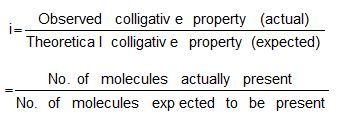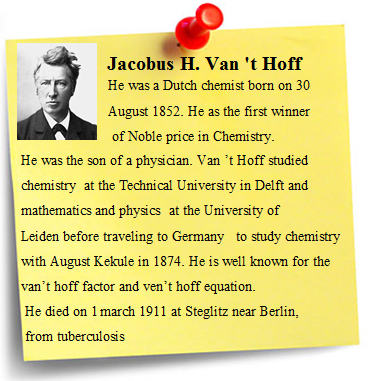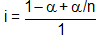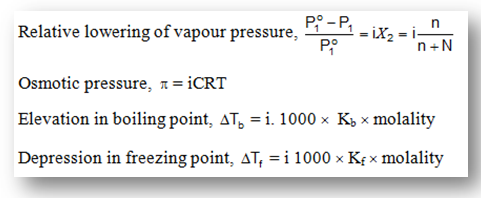×#### Thank you for registering.

One of our academic counsellors will contact you within 1 working day.

Click to Chat

1800-1023-196

+91-120-4616500

CART 0

• 0

MY CART (5)

Use Coupon: CART20 and get 20% off on all online Study Material

ITEM
DETAILS
MRP
DISCOUNT
FINAL PRICE
Total Price: Rs.

There are no items in this cart.
Continue ShoppingAbnormal colligative properties and Van't Hoff Factor

Since colligative properties depend upon the number of particles of the solute, in some cases where the solute associates or dissociates in solution, abnormal results for molecular masses are obtained.

Van't Hoff Factor :Van't Hoff, in order to account for all abnormal cases introduced a factor i known as the Van't Hoff factor, such thatAssociation :

There are many organic solutes which in non-aqueous solutions undergo association, that is, two or more molecules of the solute associate to form a bigger molecule. Thus, the number of effective molecules decreases and, consequently the osmotic pressure, the elevation of boiling point or depression of freezing point, is less than that calculated on the basis of a single molecule. Two examples are : acetic acid in benzene and chloroacetic acid in naphthalene.Association of Acetic acid in benzene through hydrogen bonding

Degree of Association :

The fraction of the total number of molecules which combine to form bigger molecule.

Consider one mole of solute dissolved in a given volume of solvent. Suppose n simple molecules combine to form an associated molecule,

i.e. nA  (A)n

Let a be the degree of association, then,

The number of unassociated moles = 1-a

The number of associated moles = a/n

Total number of effective moles = 1-a+a/ni = 1- a (1–1/n)

Obviously, i < 1

Refer to the following video for Van’t Hoff Factor

Example 1:

Question:

Acetic acid (CH3COOH) associates in benzene to form double molecules. 1.65 g of acetic acid when dissolved in 100g of benzene raised the boiling point by 0.36°C. Calculate the Van't Hoff Factor and the degree of association of acetic acid in benzene (Molal elevation constant of benzene is 2.57).

Solution:

Normal molar mass of acetic acid = 60

Observed molar mass of acetic acid.

0.508 = 1–(1–1/n) = 1 – (1–1/2) = 1–1/2

/2 = 1– 0.508 = 0.492

= 2  0.492 = 0.984

Thus acetic acid is 98.4% associated in benzene.

Dissociation

Inorganic acids, bases and salts in aqueous solutions undergo dissociation, that is, the molecules break down into positively and negatively charged ions. In such cases, the number of effective particles increases and, therefore, osmotic pressure, elevation of boiling point and depression of freezing point are much higher than those calculated on the basis of an undissociated single molecule.

Degree of Dissociation

Degree of dissociation means the fraction of the total number of molecules which dissociates in the solution, that is, breaks into simpler molecules or ions. Consider one mole of an univalent electrolyte like potassium chloride dissolved in a given volume of water. Let a be its degree of dissociation.

Then the number of moles of KCI left undissociated will be 1-a. At the same time, a moles of K+ ions and a moles of Cl-ions will be produced, as shown below.

KCl  K+ + Cl-

1-

Thus, the total number of moles after dissociation = 1-++ = 1+

Hence, i = (1+)/1

Since, as already, mentioned, osmotic pressure, vapour pressure lowering, boiling point elevation or freezing point depressions vary inversely as the molecular weight of the solute, it follows that

i = 1+  = 1+ (2–1)

In general, i = 1+ (n–1) a, Where, n = number of particles ( ions) formed after dissociation

From the above formula, it is clear that i > 1

Knowing, the observed molar mass and the Van't Hoff factor, i, the degree of dissociation, a can be easily calculated.

Now, if we include Van’t Hoff factor in the formulae for colligative properties we obtain the normal results.Note: The value of i is taken as one when solute is non electrolyte.

Example 2:

Question:

The freezing point depression constant for HgCl2 is 34.3 km–1. For a solution of 0.849 of mercurous chloride (empirical formula HgCl) in 50 g of HgCl­2, the freezing point depression is 1.24. What is the molecular weight of mercurous chloride in this solution? What is its molecular formula?

Solution:

Tf = Kf m
Molality of mercurous chloride solution = T/Kf = 0.036

M (molecular weight of mercurous chloride) = (0.849/0.036)(100/50) = 471.67

Empirical formula wt = 235.5

Molecular formula of mercurous chloride = (HgCl)2 or Hg2Cl2

Van’t Hoff Theory of Dilute Solutions

Van’t Hoff realized that an analogy exists between gases and solutions provided osmotic pressure of solutions is used in place of ordinary gas pressure. He showed that for dilute solutions of non-electrolysis the following laws hold good.

Boyle-van’t Hoff law:

The osmotic pressure (P or α) of a solution is directly proportional to its concentration (C) when the temperature is kept constant. The concentration of the solution containing one gram mole in V litres is equal to 1/V (C = 1/V)

Thus P ∝ C                 (when temperature is constant)

or P ∝ 1/V

or PV = constant

or     πV = constant

Van’t Hoff presumed that the osmotic pressure is due to the bombardment of solute molecules against the semipermeable membrane as the gas pressure is due to hits recorded by gas molecules against the walls of its container.

Pressure-Temperature law (Gay-Lussac-van’t Hoff law):

Concentration remaining same; the osmotic pressure of a dilute solution is directly proportional to its absolute temperature (T), i.e.,

P ∝ T

or P/T = constant
or
μ/T constant

Combining the two laws, i.e., when concentration and temperature both are changing, the osmotic pressure will be given by:

P ∝ CT

or P = kCT

or P = k.1/V.T           (since C = 1/V)

or PV = ST or πV = ST

S is called molar solution constant.

Here V is the volume of solution containing one gram mole of the solute. The value of 5 comes out to 0.082 lit atm K−1 mol−1 which is in agreement with the value of R, the molar gas constant. In case the solution contains n gram moles in V litres, the general equation would become  PV = nST  or     πV = ST

Third law:

Equimolecular solutions of different solutes exert equal osmotic pressure under identical conditions of temperature. Such solutions which have the same osmotic pressure are termed isotonic or iso-osmotic. When two isotonic solutions are separated by a semipermeable membrane, no flow of solvent molecules is observed on either side.

The law is similar to Avogadro’s hypothesis. It can be stated as, “Equal volumes of dilute solutions of different solutes, having the same temperature and osmotic pressure, contain equal number of molecules.”

For solution I,             PV = n1ST

For solution II,            PV = n2ST

Thus, n1 must be equal to n2 when P, V and T are same.

The analogy of dilute solutions with gases is this perfect.

This led van’t Hoff to suggest that a solute in dissolved state (i.e., in solution) behaves as a gas and the osmotic pressure of the solution is equal to the pressure which the solute would exert if it were a gas at the same temperature and occupying the same volume as that of the solution.Question 1: Total number of molecules which combine to form bigger molecule is called

a. Van't hoff factor

b. degree of dissociation

c. degree of association

d. dissociation constant

Question 2: According to Boyle-van’t Hoff law

a. πV = constant

b. i = 1+  = 1+ (2–1)

c. Tf = Kf m

d. πV = nRT

Question 3: For the reaction nA  (A)n, i =

a. 1- a (1–1/n)

b. a (1–1/n)

c. 1- (1–1/n)

d. 1- n (1–1/a)

Question 4: For association of molecules in solution

a. i = 0

b. i = 1

c. i > 1

d. i < 1Q.1

Q.2

Q.3

Q.4

c

a

a

d

Related Resources

You can also refer to the syllabus of chemistry for  IIT JEE

Look here for solved examples on solutions

To read more, Buy study materials of Solutions comprising study notes, revision notes, video lectures, previous year solved questions etc. Also browse for more study materials on Chemistry here.### Course Features

• 731 Video Lectures
• Revision Notes
• Previous Year Papers
• Mind Map
• Study Planner
• NCERT Solutions
• Discussion Forum
• Test paper with Video Solution